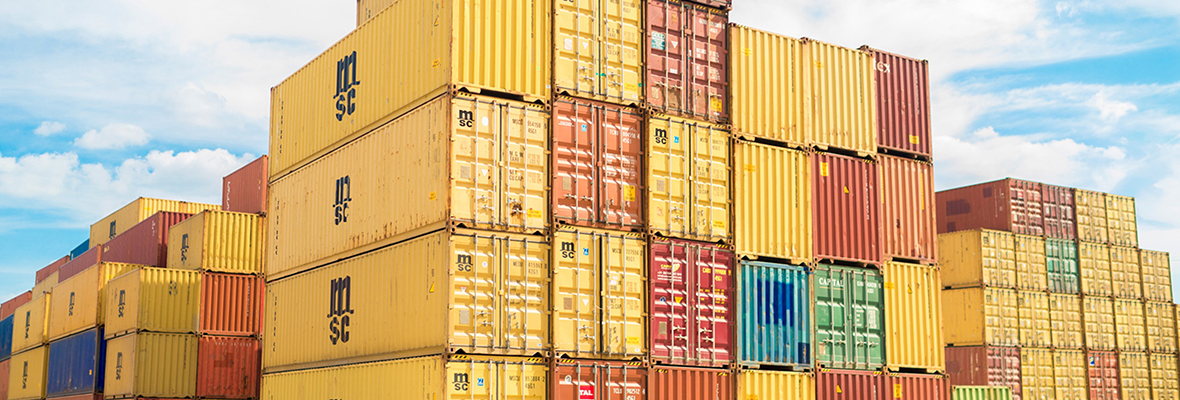+86-571-82202632                                      hito-auto@hitopec.com
You are here: / / / How to estimate the size of the container if the container data is not received?

# How to estimate the size of the container if the container data is not received?

Views:0     Author:Site Editor     Publish Time: 2019-09-29      Origin:Site

# How to estimate the size of the container if the container data is not received?

Tips for estimating container size
Many people will ask how to accurately estimate the size of the container? In fact, the method is very simple. Knowing this little trick, you don't have to wait for the factory data of the slow supply because of the size of the container.
Take a coil notebook as an example. Suppose you know that the book size is A5 size, 250gsm white card cover, 70gsm offset paper* 60 sheets.
At this time, you only know the information, and there is no real thing on hand. Even
the scale and weight of a single product are not known. How do you count it?First, first determine the individual components of the product

The A5 scale is about 21 cm * 14.8 cm

Gsm is the meaning of g/m2

Single component

= 0.21 * 0.148 * 70 * 60 + 0.21 * 0.148 * 250 * 2 + 15 = 160g

(According to the weight of the paper and the number of sheets, calculate the components of the inner page and the cover, and then estimate the weight of one coil)

Second, then determine the amount of each box

For example, the gross weight of the outer box is less than 15 kg.

We can calculate by the gross weight of 12KGS, one hundred and sixty-one thousand thousand = 75 (maybe many time customers like to pack according to the call, then 72 per box is more reasonable, of course, if you do not want to supply In the middle box, you can also directly press 48 one outer box. For details, please refer to the characteristics of your own products to decide)
container
Third, determine the carton scale
In most cases, we will know the scale of our products. In this analogy, the length and width of the notebook are known, but if you don’t know the thickness, you can only estimate it first, for example, the coil of 60 sheets. Let's press the thickness of about 6 mm. Considering that the diameter of the coil will be higher than the thickness of the book, then the time of packaging is usually two pairs of two, so we can calculate the thickness of the book by about 7 mm.
If it is 12 inner boxes, then the inner box scale is like this:
Product scale: 21cm x 14.8cm x 0.7cm
Inner box size: +1 x +1 x * 12 + 1
=:22cm x 16cm x 9.5cm

After stacking the products, the inner box size is usually 1 cm in length, width and height.

Outer box scale: (In this case, there will be 6 inner boxes in one outer box, which is assumed to be calculated by 3 layers and 2 rows)

Inner box size: 22cm x 16cm x 9.5cm
Carton size: +2 x * 2 + 2 x * 3 + 3

=:24cm x 34cm x 31.5cm

That is to say, the size of the outer box is 34 x 24cm x 31.5cm.

After the inner box is piled up, the size of the outer box is usually 2 cm in length and width, plus 3 cm in height.

Gross weight: 160 * 72 g + 2 kg inner box outer box weight = about 13.5 kgs

Remarks

1) This method is estimated after all, and it must be different from the scale and component of the test, so it is only used if the condition does not allow the test.

2) Some irregularly shaped products should be considered when considering the size of the inner box. It is reasonable to consider how the product is placed. For example, it may be necessary to consider two product pairs to save space. This time division unit scale is 2 The products are placed on the same scale to calculate.

3) If you know the scale and weight of individual products clearly, skip the first one and look at it.